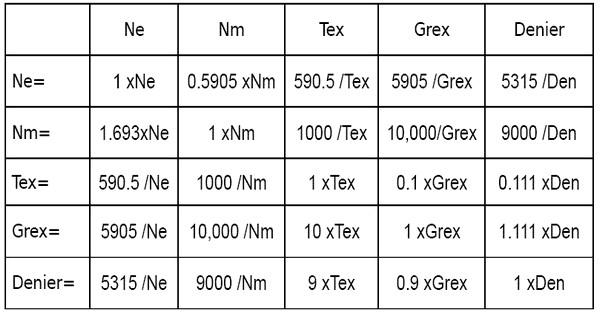## Saturday, April 1, 2017

Definition of yarn count:
Yarn count is a number, which express the fineness of coarseness of yarn. It is determined by its mass per unit length.

Yarn count measurement system:
Yarn count measurement techniques are two types.
1. Direct system
2. Indirect system
1. Direct system:
Here count is calculated by measuring weight of fixed/definite length. Tex, Denier and pounds/spyndle are the name of direct system yarn count.
 Count Name Fixed length unit Measurable weight unit Material Tex 1 km gm Filament, Yarn Denier 9 km gm All Filament Pounds/spyndle 14400 yards pounds Jute Yarn
Tex:
Weight in grams per kilometer fiber or yarn is called Tex.

Example,
If 1 km length of filament/yarn weight is 10 gm, then filament/yarn count is 10 Tex.
If 1 km length of filament/yarn weight is 25 gm, then filament/yarn count is 25 Tex.

Denier:

Weight in grams per 9 kilometer fiber or yarn is called denier.

Example,
If 9 km length of filament weight is 10 gm, then filament count is 10 Denier.
If 9 km length of filament weight is 25 gm, then filament count is 25 Denier.

Relation between Tex and Denier:

1 Denier = 9 Tex

Example,
If a filament count is 10 Tex, then this filament count is 90 Denier.
If a filament count is 20 Tex, then this filament count is 180 Denier.

Pound/Spyndle:
Weight in pounds per 14400 yards of jute yarn is called pound/spindle.

Example,
If 14400 yards length of jute yarn weight is 7 pounds, then yarn count is 7 pound/spindle.
If 14400 yards length of jute yarn weight is 9 pounds, then yarn count is 9 pound/spindle.

2. Indirect system:
Here count is calculated by measuring length of a fixed/definite weight. English (Ne), Metric (Nm), woolen and worsted are the name of indirect system yarn count.

English (Ne) count:
No of 840 yards per pounds is called English (Ne) count.

Example,
If (20x840) yards length of yarn weight is one pound than its English (Ne) count is 20.
If (45x840) yards length of yarn weight is one pound than its English (Ne) count is 45.

Metric (Nm) count:
No of kilometer per kg is called Metric (Nm) count.

Example,
If 20 kilometer length of yarn weight is one kg then its Metric (Nm) count is 20.
If 35 kilometer length of yarn weight is one kg then its Metric (Nm) count is 35.

Woolen count:
No of 256 yards per pounds is called woolen count.

Example,
If (30x256) yards length of yarn weight is one pound then its woolen count is 30.
If (45x256) yards length of yarn weight is one pound then its woolen count is 45.

Worsted (NeK) count:
No of 560 yards per pounds is called worsted count.

Example,
If (20x560) yards length of yarn weight is one pound then its worsted count is 20.
If (30x560) yards length of yarn weight is one pound then its worsted count is 30.

Linen (NeL) count:
No of 300 yards per pounds is called linen count.

Example,
If (25x300) yards length of yarn weight is one pound then its linen count is 25.
If (45x300) yards length of yarn weight is one pound then its linen count is 45.

Conversion of yarn count:
English (Ne) x Tex = 590.5
English (Ne) x Denier = 5315
English (Ne) = Metric (Nm) x 0.59
Metric (Nm) = English (Ne) x 1.7

At a glance we can see conversion of yarn count in below table:Numbering of Ply yarn:
 Direct System Indirect System NR = N x n NR = Ply yarn count (resultant count) N = Single yarn count (Tex, Denier, Pounds/spindle) n = No of yarn in ply N NR = ……           n NR = Ply yarn count (resultant count) N = Single yarn count (Ne, Nm, Woolen, Worsted, Linen) n = No of yarn in ply.
CV% means Co-efficient of variation of data. CV% count means variation of yarn count.

C.S.P. (Count Strength Product) = English count (Ne) x Lea strength in lbs.

Lea:
80 layer of yarn in which each year circumference is 1.5 yards. So, there are 80x1.5 = 120 yards in one lea.